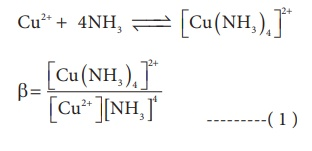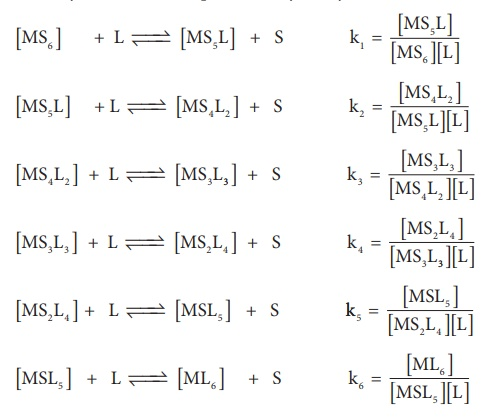Home | | Chemistry 12th Std | Stability of metal complexes

# Stability of metal complexes

The stability of coordination complexes can be interpreted in two different ways. The first one is thermodynamic stability and second one is kinetic stability.

Stability of metal complexes:

The stability of coordination complexes can be interpreted in two different ways. The first one is thermodynamic stability and second one is kinetic stability. Thermodynamic stability of a coordination complex refers to the free energy change (∆G) of a complex formation reaction. Kinetic stability of a coordination complex refers to the ligand substitution. In some cases, complexes can undergo rapid ligand substitution; such complexes are called labile complexes. However, some complexes undergo ligand substitution very slowly (or sometimes no substitution), such complexes are called inert complexes.

### Stability constant:(β)

The stability of a coordination complex is a measure of its resistance to the replacement of one ligand by another. The stability of a complex refers to the degree of association between two species involved in an equilibrium. Let us consider the following complex formation reactionSo, as the concentration of [Cu (NH3)4]2+ increases the value of stability complexes also increases. Therefore the greater the value of stability constant greater is the stability of the complex.

Generally coordination complexes are stable in their solutions; however, the complex ion can undergo dissociation to a small extent. Extent of dissociation depends on the strength of the metal ligand bond, thus Stronger the M L , lesser is the dissociation.

In aqueous solutions, when complex ion dissociates, there will be equilibrium between undissociated complex ion and dissociated ions. Hence the stability of the metal complex can be expressed in terms of dissociation equilibrium constant or instability constant α . For example let us consider the dissociation of [Cu (NH3)4 ]2+ in aqueous solution.The dissociation equilibrium constant or instability constant is represented as follows,From (1) and (2) we can say that, the reciprocal of dissociation equilibrium constant ( α) is called as formation equilibrium constant or stability constant ( β) .### Significance of stability constants

The stability of coordination complex is measured in terms of its stability constant ( β) . Higher the value of stability constant for a complex ion, greater is the stability of the complex ion. Stability constant values of some important complexes are listed in tableBy comparing stability constant values in the above table, we can say that among the five complexes listed, [Hg ( CN)4 ]2 is most stable complex ion and [ Fe ( SCN)]2+ is least stable.

## 1. Stepwise formation constants and overall formation constants

When a free metal ion is in aqueous medium, it is surrounded by (coordinated with) water molecules. It is represented as [MS6]. If ligands which are stronger than water are added to this metal salt solution, coordinated water molecules are replaced by strong ligands.

Let us consider the formation of a metal complex ML6 in aqueous medium.(Charge on the metal ion is ignored) complex formation may occur in single step or step by step.

If ligands added to the metal ion in single step, thenβoverall is called as overall stability constant. As solvent is present in large excess, its concentration in the above equation can be ignored.If these six ligands are added to the metal ion one by one, then the formation of complex [ML6] can be supposed to take place through six different steps as shown below. Generally step wise stability constants are represented by the symbol k.In the above equilibrium, the values k1 , k2 , k3 , k4 , k5 and k6 are called step wise stability constants. By carrying out small a mathematical manipulation, we can show that overall stability constant β is the product of all step wise stability constants k1 , k2 , k3 , k4 , k5 and k6 .

β = k1 × k2 × k3 × k4 × k5 × k6

On taking logarithm both sides

log ( β) = log ( k1 ) + log ( k2 ) + log ( k3 ) + log ( k4 ) + log ( k5 ) + log ( k6 )

## 2. Importance and applications of coordination complexes:

The coordination complexes are of great importance. These compounds present in many plants, animals and in minerals. Some Important applications of coordination complexes are described below.

1.        Phthalo blue – a bright blue pigment is a complex of Copper (II) ion and it is used in printing ink and in the packaging industry.

2.     Purification of Nickel by Mond’s process involves formation [Ni(CO)4], which Yields 99.5% pure Nickel on decomposition.

3.     EDTA is used as a chelating ligand for the separation of lanthanides,in softening of hard water and also in removing lead poisoning.

4.     Coordination complexes are used in the extraction of silver and gold from their ores by forming soluble cyano complex. These cyano complexes are reduced by zinc to yield metals. This process is called as Mac-Arthur –Forrest cyanide process.

5.     Some metal ions are estimated more accurately by complex formation. For example, Ni2+ ions present in Nickel chloride solution is estimated accurately for forming an insoluble complex called [Ni(DMG)2]

6.        Many of the complexes are used as catalysts in organic and inorganic reactions. For example,

(i) Wilkinson’s catalyst – [( PPh3 )3 RhCl] is used for hydrogenation of alkenes.

(ii) Ziegler-Natta catalyst - [TiCl4 ]+ Al ( C2H5 )3 is used in the polymerization of ethene.

7.        In order to get a fine and uniform deposit of superior metals (Ag, Au, Pt etc.,) over base metals, Coordination complexes [Ag ( CN)2 ] and [Au ( CN)2 ] etc., are used in electrolytic bath.

8.     Many complexes are used as medicines for the treatment of various diseases. For example,

(i) Ca-EDTA chelate, is used in the treatment of lead and radioactive poisoning. That is for removing lead and radioactive metal ions from the body.

(ii) Cis-platin is used as an antitumor drug in cancer treatment.

9.     In photography, when the developed film is washed with sodium thio sulphatesolution (hypo), the negative film gets fixed. Undecomposed AgBr forms a soluble complex called sodiumdithiosulphatoargentate(I) which can be easily removed by washing the film with water.

AgBr + 2 Na2 S2O3 → Na3 [Ag ( S 2O3 )2 ] + 2 NaBr

10. Many biological systems contain metal complexes. For example,

·           A red blood corpuscles (RBC) is composed of heme group, which is Fe2+- Porphyrin complex.it plays an important role in carrying oxygen from lungs to tissues and carbon dioxide from tissues to lungs.

·           Chlorophyll, a green pigment present in green plants and algae, is a coordination complex containing Mg2+ as central metal ion surrounded by a modified Porphyrin ligand called corrin ring. It plays an important role in photosynthesis, by which plants converts CO2 and water into carbohydrates and oxygen.

·           Vitamin B12(cyanocobalamine) is the only vitamin consist of metal ion. it is a coordination complex in which the central metal ion is Co+ surrounded by Porphyrin like ligand.

·           Many enzymes are known to be metal complexes, they regulate biological processes. For example, Carboxypeptidase is a protease enzyme that hydrolytic enzyme important in digestion, contains a zinc ion coordinated to the protein.

Tags : Coordination Chemistry | Chemistry , 12th Chemistry : UNIT 5 : Coordination Chemistry
Study Material, Lecturing Notes, Assignment, Reference, Wiki description explanation, brief detail
12th Chemistry : UNIT 5 : Coordination Chemistry : Stability of metal complexes | Coordination Chemistry | Chemistry